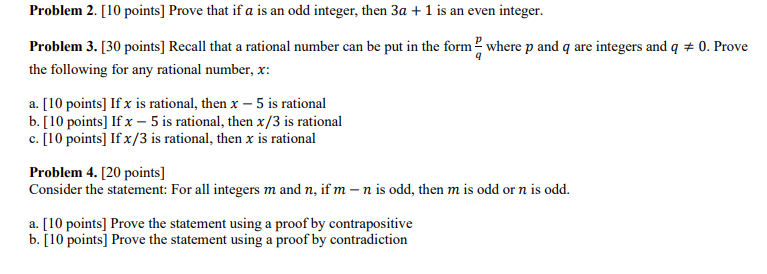# (Solved): Problem 3. [30 points] Recall that a rational number can be put in the form qp where p and q ...Problem 3. points] Recall that a rational number can be put in the form where and are integers and . Prove the following for any rational number, : a. [10 points] If is rational, then is rational b. [ 10 points] If is rational, then is rational c. [ 10 points] If is rational, then is rational Problem 4. [20 points] Consider the statement: For all integers and , if is odd, then is odd or is odd. a. [10 points] Prove the statement using a proof by contrapositive b. [10 points] Prove the statement using a proof by contradiction

We have an Answer from Expert